## Array Creation Functions

Array of ones and zeros:

ones(3) or ones(3,3) or ones([3,3])
% 3x3 matrix of all ones
ones(2,3) or ones([2,3])
% 2x3 matrix of all ones
zeros(2,3) or zeros([2,3])
% 2x3 matrix of all zeros

Identity matrix:

eye(3) or eye(3,3) or eye([3,3])
% 3x3 identity matrix
eye(2,3) or eye([2,3])
>>
1   0  0
0   1  0
eye(3,2) or eye([3,2])
>>
1   0
0   1
0   0

Random matrix (uniformly distributed):

rand(3)
% 3x3 matrix of 'uniformly distributed'
% random numbers between 0 and 1
>>  0.5668    0.0565    0.6627
0.9350    0.6745    0.1830
0.7065    0.3561    0.8112
plot(rand(1,1000),'r .')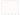hist(rand(1,1000))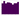Random matrix (normally distributed):

randn(3)
% 3x3 matrix of 'normally distributed' random numbers
>>
-0.1908    0.9169    0.6258
-1.8676    2.3562    0.0520
-0.1919    1.4494   -0.3593
plot(randn(1,1000),'r .')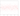X = randn(1,1000)
hist(X)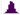hist(X,50)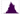Y = 5 + sqrt(10)*X
% Mean: 5, Standard deviation: sqrt(10)
hist(Y,50)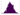Random matrices (other):

randi(5, 3)
% 3x3 matrix of 'uniformly distributed'
% pseudorandom integers between 1 and 5
>>
1   2   3
5   1   4
3   5   2
randi(5, 3, 4)
% 3x4 matrix of 'uniformly distributed'
% pseudorandom integers between 1 and 5
>>
5   2   4   1
3   5   5   5
4   1   4   5
randi([0,5], 3, 4)
% 3x4 matrix of 'uniformly distributed'
% pseudorandom integers between 0 and 5
>>
5   0   1   3
1   3   4   0
4   2   3   1
plot(randi([0,50], 1,1000),'r .')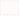hist(randi([0,50], 1,1000))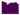Diagonal matrix:

diag([-1,2,3])
% diagonal matrix
>>
-1   0   0
0   2   0
0   0   3

Magic square:

magic(n)
% matrix constructed from the integers 1 through n^2
% with equal row and column sums. (n >= 3)
A = magic(3)
>>
8   1   6
3   5   7
4   9   2

Sum of a matrix (along some direction):

sum(A) or sum(A,1)
% column-wise sum (sum along 1st dimension)
>>  15   15   15
sum(A,2)
% row-wise sum (sum along 2nd dimension)
>>
15
15
15
sum(sum(A.*eye(3)))
% sum along main diagonal (principal diagonal, major diagonal)
>>  15
sum(sum(A.*flipud(eye(3))))
% sum along antidiagonal (secondary diagonal, minor diagonal)
>>  15

vander(v) returns the Vandermonde Matrix such that its columns are powers of the vector v.

toeplitz(c , r) returns a nonsymmetric Toeplitz matrix with c as its first column and r as its first row.

hilb(n) returns the Hilbert matrix of order n.